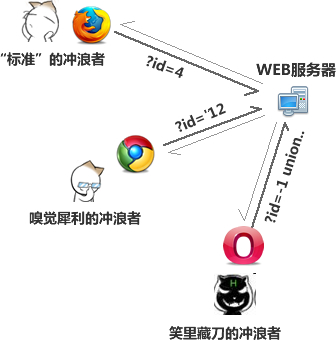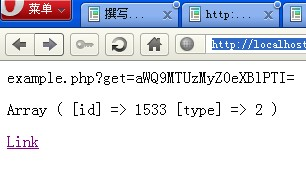WEB驱动事件与交互的最基本的方法就是通过GET参数，我想你懂paperen说的是什么意思的。

www.example.com/news/?id=234

www.example.com/goods.php?id=2web服务器来说并不知道哪些请求是恶意的是非法的，因为服务器对每个请求都是平等的，但当然不是说100个浏览者中会有99个是带恶意的，相反，恶意性的浏览者可能只有1个，但就是这么只有1个才让我们感到害怕，因为我们不知道这个带恶意的浏览者会从我们的服务器获得什么信息，发现什么漏洞……所以这也说明了为什么我们要将我们的代码写得更加稳定更加强壮，特别是应对一些异常数据的时候，这也正是为什么在发布最后要经过一个测试的原因……好吧，下面就直接奔向主题算了。

paperen认为在一定程度上你可以去屏蔽get参数的表现方式来让那些对get参数敏感的人对你失去兴趣，you know，这并不是一个很高深的方案，别怕……

www.example.com/news/?id=3&uid=45&name=paperen

www.example.com/news/?get=0qMZJevzDZaP9Wc77sh4uvhSrUXW0lYj13I4oxwNM6%

paperen想这会在安全性上更加给力，因为大部分的浏览者（或者应该说是地球人）都很难去理解这串看上去毫无意义的参数。so下面提供一下实现的方法（其实真的很简单）。想象一下自己平时是怎样处理这些get参数的。

`<?php\$id = isset( \$_GET['id'] ) ? intval( \$_GET['id'] ) : '';if ( \$id ) {    //do something}?><a href="example.php?id=164332">Link</a>`

`<?php//是否开启URL加密模式define('OPEN_URL_ENCODE', true);//加密类型define('GET_ENCODE_TYPE', 'base64');//自定义GET接受标记define('GET_TAG', 'get');//需要传递的GET参数\$args = array(    'id' => 1533,    'type' => 2,);//如果开启URL加密模式需要进行一些特殊处理if ( OPEN_URL_ENCODE ) {    \$_GET = extract_arg( \$_GET );}//整理参数function format_arg( \$url, \$args ) {    return (OPEN_URL_ENCODE) ? \$url . '?' . GET_TAG . '=' . make_my_urlarg( arg_arr2str( \$args ) ) : \$url . '?' . \$tmp;}//还原参数function extract_arg( \$arr ) {    if ( isset( \$arr[GET_TAG] ) ) {        return arg_arr2str( make_my_urlarg( \$arr[GET_TAG] , true ), true );    } else {        return array('');    }}//数组字符串互换function arg_arr2str( \$data, \$is_str2arr = false ) {    \$tmp = '';    if ( \$is_str2arr && is_string( \$data ) ) {        \$arr = explode('&', \$data);        foreach( \$arr as \$index => \$data ) {            if ( false !== strpos(\$data, '=') ) {                list(\$k , \$val) = explode('=', \$data);                \$tmp[\$k] = \$val;            }        }    } else if( !\$is_str2arr && is_array( \$data ) ) {        foreach( \$data as \$k => \$val ) \$tmp .= '&' . \$k . '=' . \$val;        \$tmp = substr(\$tmp, 1);    }    return \$tmp;}//根据相应的算法加解密参数function make_my_urlarg(\$str, \$is_decode = false) {    if ( 'base64' == GET_ENCODE_TYPE ) {        return (\$is_decode) ? base64_decode(\$str) : base64_encode(\$str);    }}//还是一如既往地接收GET参数，感觉这个加解密处理与我们无关\$id = isset( \$_GET['id'] ) ? intval(\$_GET['id']) : '';\$type = isset( \$_GET['type'] ) ? intval(\$_GET['type']) : '';if ( \$id ) {    //do something}\$url = format_arg('example.php', \$args);echo "<p>\$url</p>";print_r( \$_GET );echo '<p></p>';?><a href="<?php echo \$url; ?>">Link</a>``/** * authcode 摘自UChome * @param string \$string 明文 * @param string \$operation ENCODE加密/DECODE解密 * @param string \$key 密钥（可以在配置文件中定义） * @param string \$expiry 有效期？ * @return string */function authcode(\$string, \$operation = 'DECODE', \$key = '', \$expiry = 0) {    \$ckey_length = 1;    \$key = md5(\$key ? \$key : '');    \$keya = md5(substr(\$key, 0, 16));    \$keyb = md5(substr(\$key, 16, 16));    \$keyc = \$ckey_length ? (\$operation == 'DECODE' ? substr(\$string, 0, \$ckey_length): substr(md5(microtime()), -\$ckey_length)) : '';    \$cryptkey = \$keya . md5(\$keya.\$keyc);    \$key_length = strlen(\$cryptkey);    \$string = \$operation == 'DECODE' ? base64_decode(substr(\$string, \$ckey_length)) : sprintf('%010d', \$expiry ? \$expiry + time() : 0).substr(md5(\$string.\$keyb), 0, 16).\$string;    \$string_length = strlen(\$string);    \$result = '';    \$box = range(0, 255);    \$rndkey = array();    for(\$i = 0; \$i <= 255; \$i++) {        \$rndkey[\$i] = ord(\$cryptkey[\$i % \$key_length]);    }    for(\$j = \$i = 0; \$i < 256; \$i++) {        \$j = (\$j + \$box[\$i] + \$rndkey[\$i]) % 256;        \$tmp = \$box[\$i];        \$box[\$i] = \$box[\$j];        \$box[\$j] = \$tmp;    }    for(\$a = \$j = \$i = 0; \$i < \$string_length; \$i++) {        \$a = (\$a + 1) % 256;        \$j = (\$j + \$box[\$a]) % 256;        \$tmp = \$box[\$a];        \$box[\$a] = \$box[\$j];        \$box[\$j] = \$tmp;        \$result .= chr(ord(\$string[\$i]) ^ (\$box[(\$box[\$a] + \$box[\$j]) % 256]));    }    if(\$operation == 'DECODE') {        if((substr(\$result, 0, 10) == 0 || substr(\$result, 0, 10) - time() > 0) && substr(\$result, 10, 16) == substr(md5(substr(\$result, 26).\$keyb), 0, 16)) {            return substr(\$result, 26);        } else {            return '';        }    } else {        return \$keyc.str_replace('=', '', base64_encode(\$result));    }}`

I AM HERE!这里